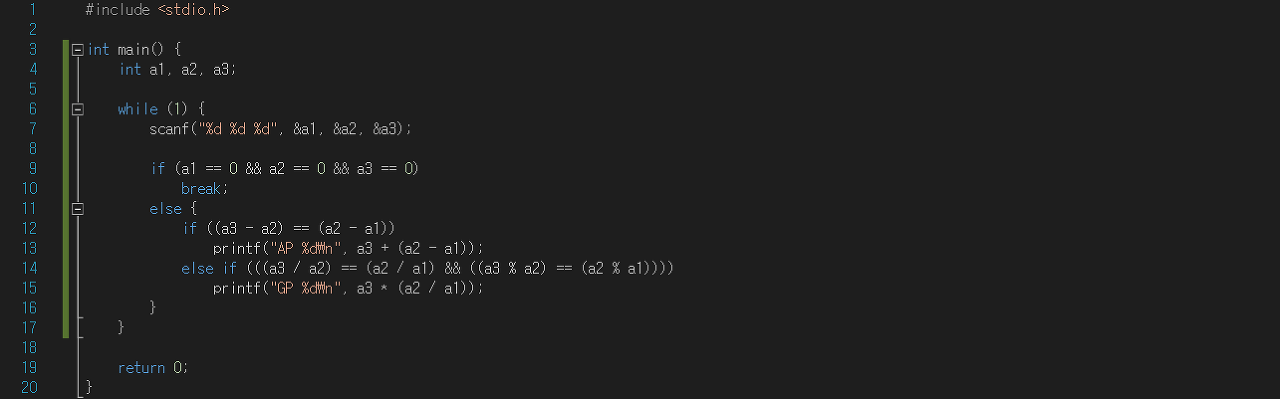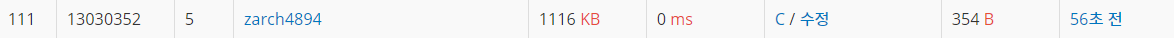# [백준] 4880 - What's Next?### 문제 정보

• 문제 이름: What’s Next? (원문)
• 문제 번호: 4880
• 문제 유형: -
• 풀이 언어: `C`
• 제출 시간: 2019년 5월 4일 17시 25분 44초
• 원본 링크: https://www.acmicpc.net/problem/4880

### 문제

According to Wikipedia, an arithmetic progression (AP) is a sequence of numbers such that the diﬀerence of any two successive members of the sequence is a constant. For instance, the sequence 3, 5, 7, 9, 11, 13, . . . is an arithmetic progression with common diﬀerence 2. For this problem, we will limit ourselves to arithmetic progression whose common diﬀerence is a non-zero integer.

On the other hand, a geometric progression (GP) is a sequence of numbers where each term after the ﬁrst is found by multiplying the previous one by a ﬁxed non-zero number called the common ratio. For example, the sequence 2, 6, 18, 54, . . . is a geometric progression with common ratio 3. For this problem, we will limit ourselves to geometric progression whose common ratio is a non-zero integer.

Given three successive members of a sequence, you need to determine the type of the progression and the next successive member.

### 입력

Your program will be tested on one or more test cases. Each case is speciﬁed on a single line with three integers (−10,000 < a1, a2, a3 < 10,000) where a1, a2, and a3 are distinct. The last case is followed by a line with three zeros.

### 출력

For each test case, you program must print a single line of the form: XX┐ v

where XX is either AP or GP depending if the given progression is an Arithmetic or Geometric Progression. v is the next member of the given sequence. All input cases are guaranteed to be either an arithmetic or geometric progressions.

### 결과 - 소스코드 및 랭크### 메모

1년 전에 틀렸던 문제였는데 오늘 다시 시도해보았다.

전에 이 문제를 풀 때 분명 쉬운 문제인데 왜 안 풀리지 고민을 하면서 여러 번 제출을 했었는데 이제와서 다시 보니 등비수열을 이루는 부분에서 몫만 신경쓰고 나머지 값은 생각하지 않고 있었던 것이었다.

그래서 그 경우를 추가하여 문제를 해결할 수 있었다.

Alec J

2019-05-04

2022-04-07